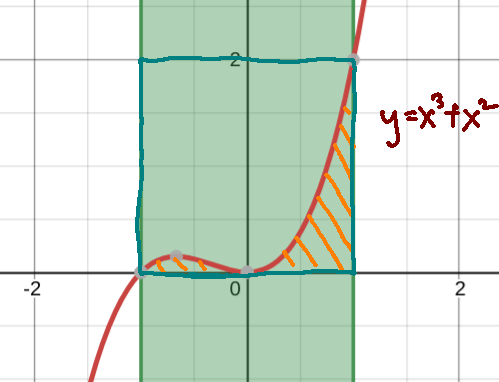#### Let’s solve Integration problems with monte carlo sampling!

Apart from the above intuitive method of deducing the value of pi simply from the shape of a circle within a square, there are other useful applications of monte carlo sampling. For instance, we can sample repeatedly to deduce the area under the graph , ie. the integral of a function.

Problem: Evaluate the definite integral of the function$x^3+x^2$ for$-1\le x \le 1$
This is shown by the area shaded in orange as shown in this image.We can create a “box” to perform monte carlo sampling with UniformDistributionOverBox which takes in a Real Interval, with dimensions (of the cyan box) as shown in the image above

To define Polynomials in s2, we use the following
Polynomial(3.0,5.0,0.0,0.0) will define the following polymonial$3x^3+5x^2$

Some useful associate methods are available here :

• To get the corresponding result when x value is placed into the polynomial, we can use .evaluate(x) where x is the corresponding x value and  is the polynomial defined earlier saved as a variable
• We can also print the degree of polynomial (aka the power of the term with the largest effect on the outcome of the polynomial) via:
• .degree()
• We can get the coefficients of the various terms with :
• .getCoefficients() for instance for the first term